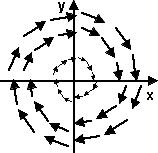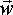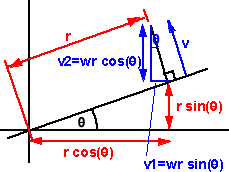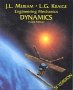# Using Vector Calculus to analyse rotation of solid objects

Vector Calculus is often used to analyse the movement of fluids, but there is no reason why we should not use it to analyse solid objects, provided that we apply it to regons of space where the vector field is continous..

If we take a velocity field of a rotating object we might get a field that looks like this:If we take the curl of this field we would get a different vector fieldTo see how this relates to the angular velocity () we can calculate curl as follows, if the following diagram shows the velocity of a point on a solid object rotating about the origin:v1 = component of v in x dimention = -v sin(θ) = -wr sin(θ)
v2 = component of v in y dimention = v cos(θ) = wr cos(θ)
v3 = component of v in z dimention = 0

x = r cos(θ)
y = r sin(θ)
z = 0

dv1/dy = d(-wr sin(θ)/d(r sin(θ)) = -w
dv1/dz = 0 /* because the object boes not change in the z direction */
dv2/dx = d(wr cos(θ)/d(r cos(θ)) = w
dv2/dz = 0
dv3/dx = 0 /* because there is no component of velocity in the z direction */
dv3/dy = 0

so:

curl() = (d v3 / dy - d v2 / dz)i + (d v1 / dz - d v3 / dx)j + (d v2 / dx - d v1 / dy)k

= 0 i + 0 j + (w + w) k

= 2w k

Therefore curl of a solid object is 2w along the line of the axis of rotation.

 metadata block see also: Correspondence about this page Open Forum - w x r or r x w ? Mati Mr. Dual Space Book Shop - Further reading. Where I can, I have put links to Amazon for books that are relevant to the subject, click on the appropriate country flag to get more details of the book or to buy it from them.Engineering Mechanics Vol 2: Dynamics - Gives theory for rigid dynamics, aims to allow prediction of effects of force and motion. Includes rotating frame of reference. Lots of colour diagrams, I guess its college / University level. Commercial Software Shop Where I can, I have put links to Amazon for commercial software, not directly related to the software project, but related to the subject being discussed, click on the appropriate country flag to get more details of the software or to buy it from them.Mathmatica

This site may have errors. Don't use for critical systems.